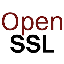OpenSSL "ans1parse" - Wrap of SEQUENCE Structure

Q

What is the wrap of an ASN.1 SEQUENCE structure?

✍: FYIcenter.com

AThe wrap of an ASN.1 SEQUENCE structure is the binary string of ASN.1 SEQUENCE structure in DER format stored in an BIT STRING or OCTET STRING field in another ASN.1 SEQUENCE structure.

The main purpose of using the SEQUENCE wrap is to make the SEQUENCE structure invisible in the enclosing SEQUENCE.

For example, we have the following nested SEQUENCE structure:

```Question SEQUENCE:{
trackingNumber = INTEGER:5
questionText   = IA5STRING:"In asymmetric encryption, which key can be given out?"
optionA = IA5STRING:"A) Private key"
optionB = IA5STRING:"B) Public key"
optionC = IA5STRING:"C) Both keys"
}
}
```

If we want to make answerOption SEQUENCE invisible, we can BIT STRING to wrap it:

```Question SEQUENCE:{
trackingNumber = INTEGER:5
questionText   = IA5STRING:"In asymmetric encryption, which key can be given out?"
answerOption   = BITSTRING: (DER binary string of answerOption)
}
```

The test below is a demonstration of storing a SEQUENCE wrap as a BIT STRING:

```C:\Users\fyicenter>type asn1_question_option.cnf
asn1 = SEQUENCE:optionSection

[optionSection]
optionA = IA5STRING:"A) Private key"
optionB = IA5STRING:"B) Public key"
optionC = IA5STRING:"C) Both keys"

C:\Users\fyicenter>\local\openssl\openssl asn1parse
-genconf asn1_question_option.cnf -out question_option.der
0:d=0  hl=2 l=  45 cons: SEQUENCE
2:d=1  hl=2 l=  14 prim: IA5STRING         :A) Private key
18:d=1  hl=2 l=  13 prim: IA5STRING         :B) Public key
33:d=1  hl=2 l=  12 prim: IA5STRING         :C) Both keys

C:\Users\fyicenter>bin2hex question_option.der test.hex
302d160e41292050726976617465206b6579160d4229205075626c6963206b65
79160c432920426f7468206b657973

C:\Users\fyicenter>type asn1_question_wrap.cnf
asn1 = SEQUENCE:questionSection

[questionSection]
trackingNumber = INTEGER:5
questionText   = IA5STRING:"In asymmetric encryption, which key can be given out?"
6579160d4229205075626c6963206b6579160c432920426f7468206b657973

C:\Users\fyicenter>\local\openssl\openssl asn1parse
-genconf asn1_question_wrap.cnf -out question_wrap.der
0:d=0  hl=2 l= 108 cons: SEQUENCE
2:d=1  hl=2 l=   1 prim: INTEGER           :05
5:d=1  hl=2 l=  53 prim: IA5STRING         :In asymmetric encryption,
which key can be given out?
60:d=1  hl=2 l=  48 prim: BIT STRING

C:\Users\fyicenter>\local\openssl\openssl asn1parse -genc
onf asn1_question_wrap.cnf -out question_wrap.der -strparse 60
0:d=0  hl=2 l=  45 cons: SEQUENCE
2:d=1  hl=2 l=  14 prim: IA5STRING         :A) Private key
18:d=1  hl=2 l=  13 prim: IA5STRING         :B) Public key
33:d=1  hl=2 l=  12 prim: IA5STRING         :C) Both keys
```

Note that "asn1parse -strparse 60" command is smart to know that the BIT STRING field at 0x60 offset is a SEQUENCE wrap.

2016-09-28, 5512👍, 0💬